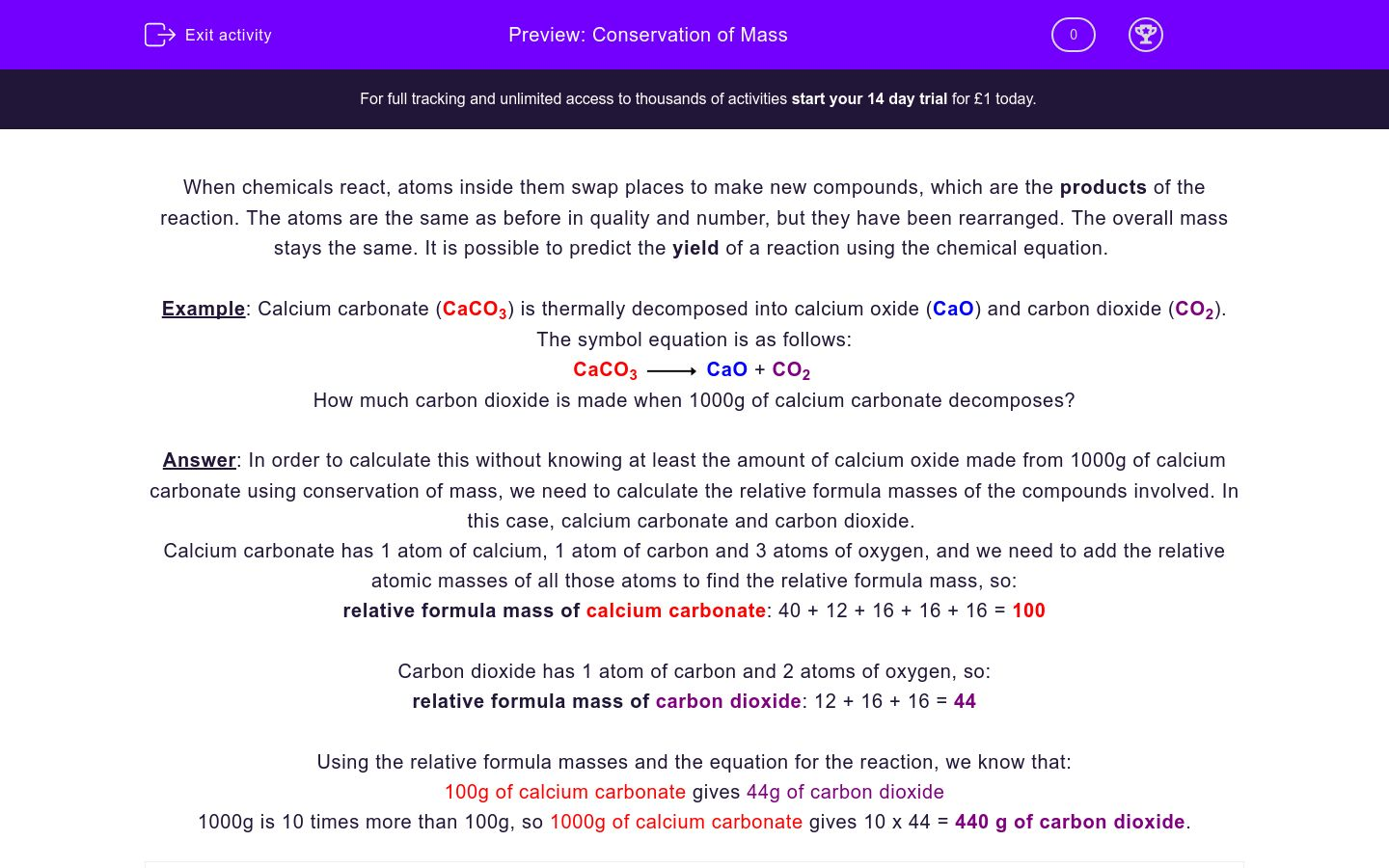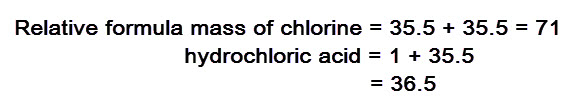# Conservation of Mass

In this worksheet, students consider mass, which is conserved in chemical reactions meaning that the mass of the reactants is equal to the mass of the products and relative formula masses can be used to predict the yield of reactions.Key stage:  KS 4

Curriculum topic:  Chemistry: Atomic Structure and the Periodic Table

Curriculum subtopic:  Chemical Reactivity of Elements

Difficulty level:### QUESTION 1 of 10

When chemicals react, atoms inside them swap places to make new compounds, which are the products of the reaction. The atoms are the same as before in quality and number, but they have been rearranged. The overall mass stays the same. It is possible to predict the yield of a reaction using the chemical equation.

Example: Calcium carbonate (CaCO3) is thermally decomposed into calcium oxide (CaO) and carbon dioxide (CO2). The symbol equation is as follows:

CaCO3CaO + CO2

How much carbon dioxide is made when 1000g of calcium carbonate decomposes?

Answer: In order to calculate this without knowing at least the amount of calcium oxide made from 1000g of calcium carbonate using conservation of mass, we need to calculate the relative formula masses of the compounds involved. In this case, calcium carbonate and carbon dioxide.

Calcium carbonate has 1 atom of calcium, 1 atom of carbon and 3 atoms of oxygen, and we need to add the relative atomic masses of all those atoms to find the relative formula mass, so:

relative formula mass of calcium carbonate: 40 + 12 + 16 + 16 + 16 = 100

Carbon dioxide has 1 atom of carbon and 2 atoms of oxygen, so:

relative formula mass of carbon dioxide: 12 + 16 + 16 = 44

Using the relative formula masses and the equation for the reaction, we know that:

100g of calcium carbonate gives 44g of carbon dioxide

1000g is 10 times more than 100g, so 1000g of calcium carbonate gives 10 x 44 = 440 g of carbon dioxide.

This is the symbol equation for the thermal decomposition of calcium carbonate:

CaCO3CaO + CO

How much calcium oxide is produced from 500g of calcium carbonate? Type the number of grams (just write the number).

Relative atomic mass of calcium: 40, carbon: 12, oxygen: 16

Hydrogen and chlorine produce hydrochloric acid:

H2 + Cl22HCl

How much HCl acid is made from 6g of hydrogen? Type your answer in grams (just write the number).

Relative atomic mass of hydrogen = 1 and chlorine = 35.5

Propane burns and gives carbon dioxide and water:

C3H8 + 5O23CO2 + 4H2O

How many grams of water are produced when 22 g of propane (C3H8) burn? (Just write the number).

Relative atomic mass of carbon = 12, hydrogen = 1, oxygen = 16

Hydrogen and chlorine produce hydrochloric acid:

H2 + Cl22HCl

How much HCl acid is made from 35.5 g of chlorine? Type your answer in grams (just type the number).

Relative atomic mass of hydrogen = 1, chlorine = 35.5

propane burns with oxygen to produce carbon dioxide and water:

C3H8 + 5O3CO2 + 4H2O

How many grams of carbon dioxide are produced when 88 g of propane (C3H8) burn? (just write the number).

Relative atomic mass of carbon = 12, hydrogen = 1, oxygen = 16

What is the maximum total mass (in grams) produced from 100g of reactants?

1g

50g

99g

100g

This is a general chemical equation for when reactants A and B give products C and D.

A + BC + D

mass of A = 2g

mass of B = 3g

mass of C = 1g

Use the above information to work out the mass of D. Remember, the mass of the products is equal to the mass of reactants.

1g

2g

3g

4g

Calculate how many grams of carbon monoxide are formed from 6g of carbon.

2C + O22CO

Relative atomic mass of C = 12, O = 16

(just write the number in the answer box below).

Why is mass conserved in chemical reactions?

the number of atoms changes

the number of molecules is the same

the number of atoms remains the same but they just swap places

In an industrial reaction, 20g of a wanted substance was expected to be produced, but instead only 10g was produced. What was the percentage yield?

45%

50%

55%

• Question 1

This is the symbol equation for the thermal decomposition of calcium carbonate:

CaCO3CaO + CO

How much calcium oxide is produced from 500g of calcium carbonate? Type the number of grams (just write the number).

Relative atomic mass of calcium: 40, carbon: 12, oxygen: 16

CORRECT ANSWER
280
EDDIE SAYS
relative formula mass of calcium carbonate = 40 + 12 + 16 + 16 + 16 = 100
relative atomic mass of calcium oxide = 40 + 16 = 56
100g of calcium carbonate gives 56g of calcium oxide
500g would give 5 times more, 56 x 5 = 280g calcium oxide
• Question 2

Hydrogen and chlorine produce hydrochloric acid:

H2 + Cl22HCl

How much HCl acid is made from 6g of hydrogen? Type your answer in grams (just write the number).

Relative atomic mass of hydrogen = 1 and chlorine = 35.5

CORRECT ANSWER
219
EDDIE SAYS2g of hydrogen gives 2 x 36.5 = 73 g of HCl
You have to multiply by 2 because of the 2 in front of HCl in the equation, which shows that 2 molecules of HCl are produced.
6g of hydrogen would give 3 times more (6/2=3), therefore 3 x 73 = 219 g of HCl
• Question 3

Propane burns and gives carbon dioxide and water:

C3H8 + 5O23CO2 + 4H2O

How many grams of water are produced when 22 g of propane (C3H8) burn? (Just write the number).

Relative atomic mass of carbon = 12, hydrogen = 1, oxygen = 16

CORRECT ANSWER
36
EDDIE SAYS
Relative formula mass of propane = 12 + 12 + 12 + 1 + 1 + 1 + 1 + 1 + 1 + 1 + 1 = 44
Relative atomic mass of water = 1 + 1 + 16 = 18, but 4 molecules of water are produced: 18 x 4 = 72
44 g of propane give 72 g of water
22 g is half of 44 so it gives 72/2 = 36 g of water
• Question 4

Hydrogen and chlorine produce hydrochloric acid:

H2 + Cl22HCl

How much HCl acid is made from 35.5 g of chlorine? Type your answer in grams (just type the number).

Relative atomic mass of hydrogen = 1, chlorine = 35.5

CORRECT ANSWER
36.5
EDDIE SAYS71 g of chlorine give 2 times 36.5 = 73 g of HCl
You have to multiply by 2 because of the 2 in front of HCl in the equation, which shows that 2 molecules of HCl are produced.
35.5 g of chlorine would give half this amount (71/35.5=2), therefore 73/2 = 36.5 g of HCl
• Question 5

propane burns with oxygen to produce carbon dioxide and water:

C3H8 + 5O3CO2 + 4H2O

How many grams of carbon dioxide are produced when 88 g of propane (C3H8) burn? (just write the number).

Relative atomic mass of carbon = 12, hydrogen = 1, oxygen = 16

CORRECT ANSWER
264
EDDIE SAYS
Relative formula mass of propane = 12 + 12 + 12 + 1 + 1 + 1 + 1 + 1 + 1 + 1 + 1 = 44
Relative atomic mass of carbon dioxide = 12 + 16 + 16 = 44, but 3 molecules of carbon dioxide are produced: 3 x 44 = 132
44 g of propane give 132 g of carbon dioxide
88 g is double 44 so it gives 132 x 2 = 264 g of carbon dioxide
• Question 6

What is the maximum total mass (in grams) produced from 100g of reactants?

CORRECT ANSWER
100g
EDDIE SAYS
The law of conservation of mass states that the mass of reactants is equal to the mass of products, so 100 g of products would be given.
• Question 7

This is a general chemical equation for when reactants A and B give products C and D.

A + BC + D

mass of A = 2g

mass of B = 3g

mass of C = 1g

Use the above information to work out the mass of D. Remember, the mass of the products is equal to the mass of reactants.

CORRECT ANSWER
4g
EDDIE SAYS
mass of reactants = 2 + 3 = 5g
mass of reactants = mass of products = mass of C + mass of D = 5 g
mass of C = 1 g, so mass of D = 5 - 1 = 4 g
• Question 8

Calculate how many grams of carbon monoxide are formed from 6g of carbon.

2C + O22CO

Relative atomic mass of C = 12, O = 16

(just write the number in the answer box below).

CORRECT ANSWER
14
EDDIE SAYS
2 x 12 = 24 g of carbon give 2 x (12 + 16) = 56 g of carbon monoxide
24/6 = 4 times less carbon monoxide would be produced by 6 g of carbon, so 56/4 = 14 g of carbon monoxide are produced.
• Question 9

Why is mass conserved in chemical reactions?

CORRECT ANSWER
the number of atoms remains the same but they just swap places
EDDIE SAYS
The atoms are the same as before in quality and number, they are just rearranged. The overall mass stays the same.
• Question 10

In an industrial reaction, 20g of a wanted substance was expected to be produced, but instead only 10g was produced. What was the percentage yield?

CORRECT ANSWER
50%
EDDIE SAYS
10g is half of 20g, so 50% was produced.
---- OR ----

Sign up for a £1 trial so you can track and measure your child's progress on this activity.

### What is EdPlace?

We're your National Curriculum aligned online education content provider helping each child succeed in English, maths and science from year 1 to GCSE. With an EdPlace account you’ll be able to track and measure progress, helping each child achieve their best. We build confidence and attainment by personalising each child’s learning at a level that suits them.

Get started#### Similar activities you may like

Start your £1 trial today.
Subscribe from £10/month.